• 这篇文章是基于bilibili的吴恩达机器学习经典名课【中英字幕】所写的第三周的编程练习，这个作业是关于逻辑回归的。 作业大纲1 逻辑回归1.1 数据可视化1.2 具体实现过程1.2.1 热身练习：sigmoid函数sigmoid.m1.2.2 ...
这篇文章是基于bilibili的吴恩达机器学习经典名课【中英字幕】所写的第三周的编程练习，这个作业是关于逻辑回归的。
作业大纲1 逻辑回归1.1 数据可视化1.2 具体实现过程1.2.1 热身练习：sigmoid函数sigmoid.m1.2.2 代价函数梯度 costFunction.m1.2.3 使用fminunc函数调参1.2.4 评估逻辑回归 predict.m2 正则化逻辑回归2.1 数据可视化2.2 特征映射 mapFeature.m2.3 代价函数和梯度 costFunctionReg.m2.3.1 使用fminunc函数调参2.4 画出决策边界2.5 选做练习总结
1 逻辑回归
这是一个预测学生能否被大学录取的二分类问题。问题给了学生两门考试的成绩以及能否被录取的结果。在这个部分，有一个ex2.m的主函数，以及辅助运行需要你去完成的子函数。
1.1 数据可视化
就是将两门成绩画在平面坐标轴上，然后是否录取要分开标识出来，并且将plotData.m函数补充完整。
function plotData(X, y)
figure; hold on;
pos = find(y==1); neg = find(y==0);
plot(X(pos,1),X(pos,2),'k+','LineWidth',2,'MarkerSize', 7);
plot(X(neg, 1), X(neg, 2), 'ko', 'MarkerFaceColor', 'y', 'MarkerSize', 7);
hold off;
end
画出来的图像是这样的：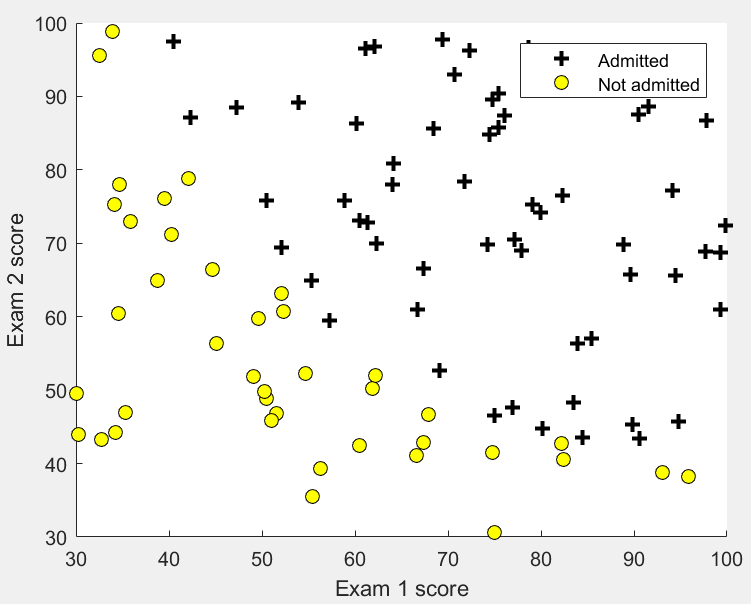1.2 具体实现过程
1.2.1 热身练习：sigmoid函数sigmoid.m
这个部分是要我们完成sigmoid函数的表示方法，同时要支持向量，这个就是对于输入的z的每一个值都计算他的sigmoid值就可以了，那就是用向量的点乘即可。
function g = sigmoid(z)
g = 1 ./ (1 + exp(-z));
end
1.2.2 代价函数梯度 costFunction.m
给出逻辑回归的代价函数，以及各个梯度的公式，要求你在 costFunction.m 这个函数中，将这两个公式进行实现，需要注意的是，这些变量都是矩阵之间的计算。
代价函数：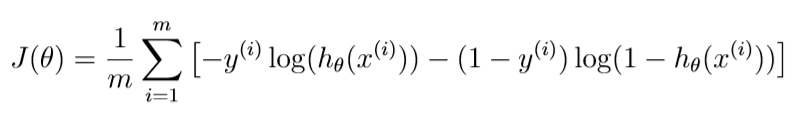梯度：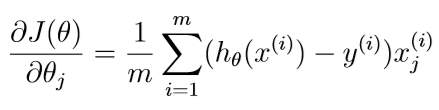function [J, grad] = costFunction(theta, X, y)m = length(y); % number of training examples
h = sigmoid(X*theta);
J = (-y'*log(h)-(1-y)'*log(1-h))/m;
grad = X'*(h-y)/m;
% =============================================================
end
1.2.3 使用fminunc函数调参
这个就不用做什么，老师把代码都写在ex2.m这个函数里面。就是使用fminunc这个函数，并且调用你自己写的costFunction.m就可以了。
1.2.4 评估逻辑回归 predict.m
对于自己已经选好的最合适的参数，怎么去检验这个模型的好坏呢？应用自己的模型在训练集上进行测试，看看预测的准确率。完成predict.m函数。
function p = predict(theta, X)
p = sigmoid(X*theta);
n = length(p);
for i=1:n
if p(i)>=0.5
p(i) = 1;
else
p(i) = 0;
end
end
% =========================================================================
end
2 正则化逻辑回归
对于正则化，就是为了避免所选的模型过拟合overfit，过拟合就是指在训练集样本上拟合得很好，但是其泛化能力很差，即不能有效地应用在测试样本上。正则化就是在原有的代价函数上增加惩罚项，使所选取的参数不能无限制的大。
在这个部分，给到的是预测制造工厂的微芯片是否通过质量保证（QA），训练集还是为两个测试的分数，以及能否通过的0-1变量。这个部分的主函数是ex2 reg.m 。
2.1 数据可视化
还是一样，拿到数据就尽量可视化在坐标轴上面。这个部分都不用改，直接用上面的plotData函数即可。得到的图形：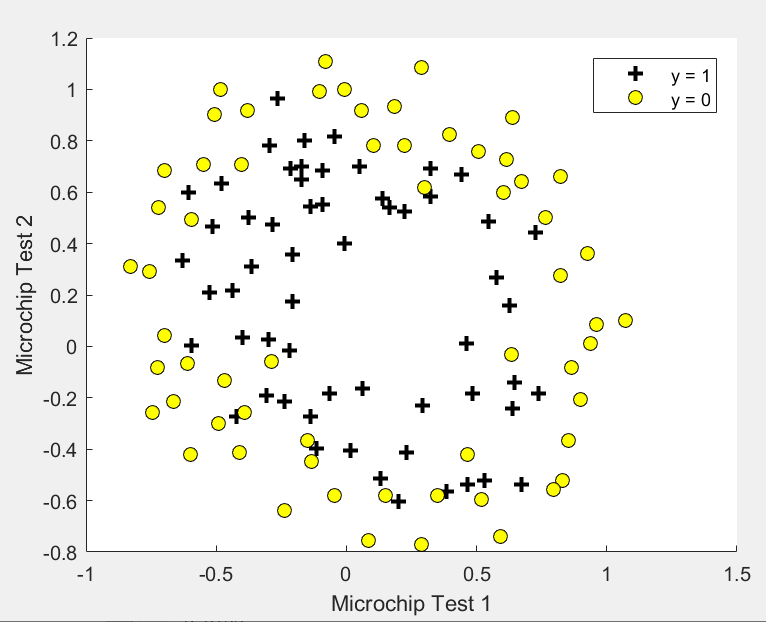从图上就可以看出来，这次的模型一定会比较复杂一些。
2.2 特征映射 mapFeature.m
对于更好拟合这个问题，我们可以采用增加特征的方法。在已经给出的mapFeature.m这个函数中，将x1和x2的所有多项式项都升幂到了6次。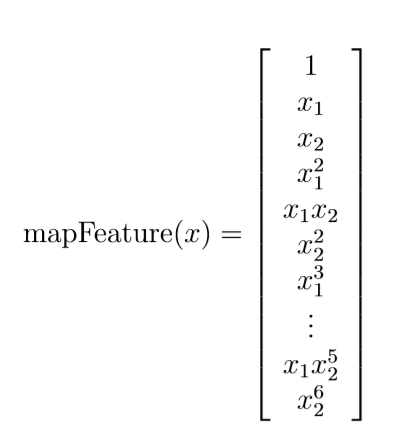一共有28项，（1+7）*7/2=28.
2.3 代价函数和梯度 costFunctionReg.m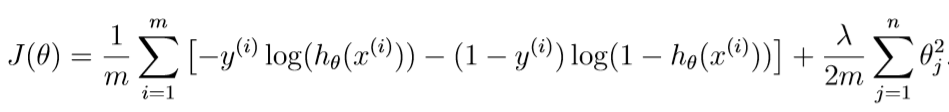这个就是加了正则项的代价函数。
梯度：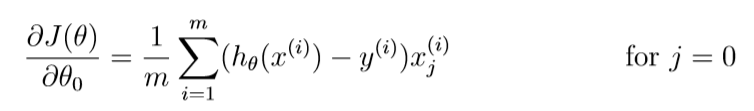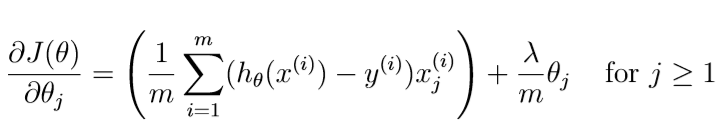注意这里的theta(0)要分开写。
2.3.1 使用fminunc函数调参
老师把代码都写在ex2_reg.m这个函数里面。就是使用fminunc这个函数，并且调用你自己写的costFunctionReg.m就可以了。
2.4 画出决策边界
也不用自己动手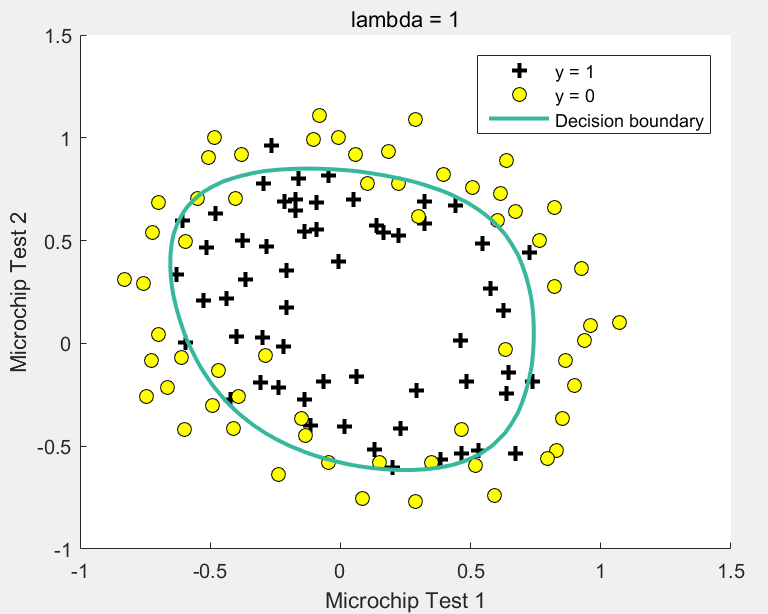2.5 选做练习
就是改变正则化参数λ的值，看看边界会发生什么变化，我这里就不演示啦。
总结
其实我觉得老师布置的题目还是少了一点力度，就是说老师帮我们做的太多啦。但是对于这种要上交评分的作业，可能是要规定一些格式吧，能做到这样子已经很棒啦。
我也是在上了老师的课之后才真正地了解到一些自己在用的算法到底是什么做到的，总之就是很有意思的东西。
我的成绩：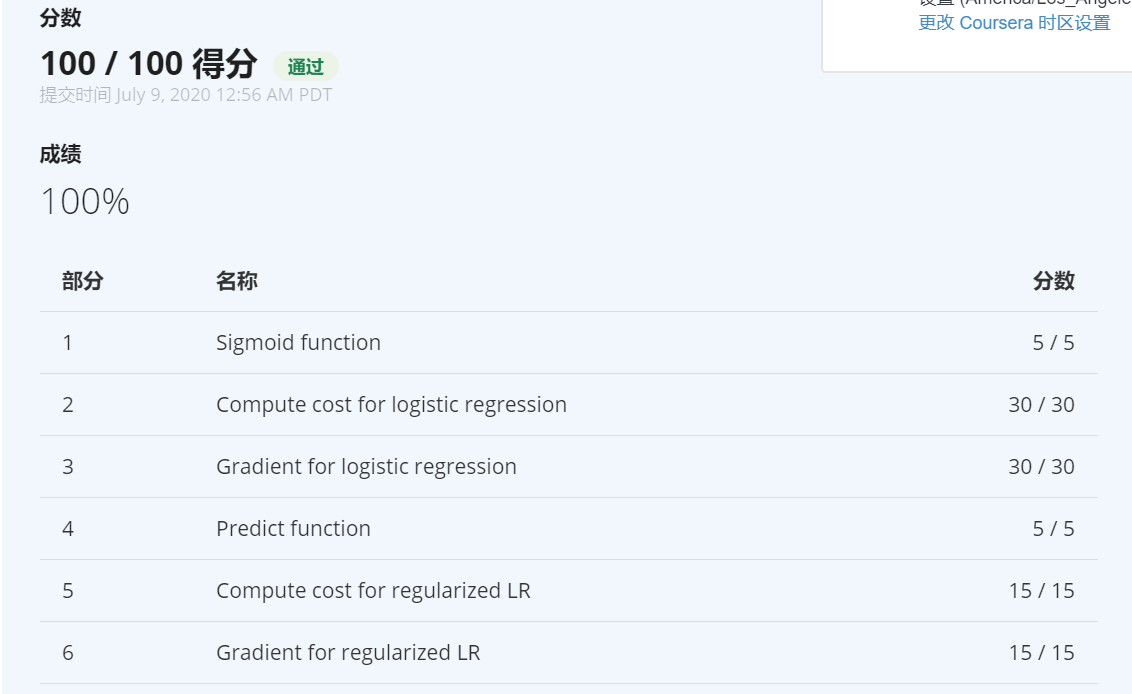展开全文逻辑回归
• 本文基于吴恩达老师第五周的练习，在这次的练习中，你将会实现神经网路的后向传播算法(BP神经网络)。 作业大纲1 神经网络 Neural Networks ex4.m1.1 数据可视化1.2 模型表示1.3 前向传播和代价函数 nnCostFunction.m...
本文基于吴恩达老师第五周的练习，在这次的练习中，你将会实现神经网路的后向传播算法(BP神经网络)。
作业大纲1 神经网络 Neural Networks ex4.m1.1 数据可视化1.2 模型表示1.3 前向传播和代价函数 nnCostFunction.m1.4 正则化代价函数2 后向传播 nnCostFunction.m2.1 Sigmoid函数的导数 sigmoidGradient.m2.2 随机初始化 randInitializeWeights.m2.3 后向传播梯度检查2.5 正则化神经网络2.6 使用fmincg来学习参数3 隐层可视化总结
1 神经网络 Neural Networks ex4.m
在以前的练习中，你实现了神经网络的前向传播并且用已知的权重进行手写数字的预测。在这次的练习中，你将实现后向传播算法进行参数的学习。
1.1 数据可视化
就和上周一样展示数据，有5000个样本，一个样本就是一张手写数字的图片，20*20px，有400个特征。然后y中，0用10来表示，其他数字不变。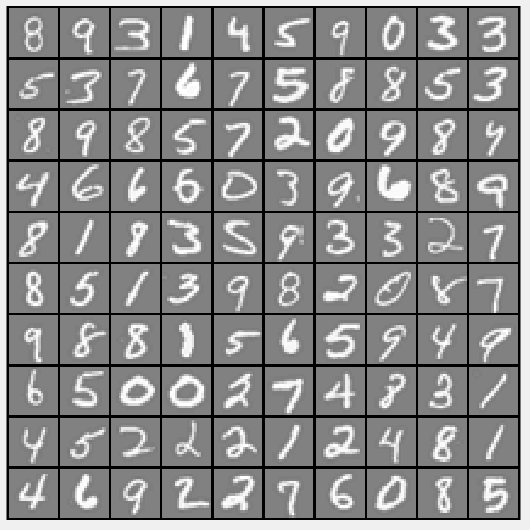1.2 模型表示
本次训练的模型是一个三层的神经网络，一个输入层，有400个单元。一个隐含层，有25个单元。一个输出层，有十个单元，分别对应了十个数字类别。
1.3 前向传播和代价函数 nnCostFunction.m
现在你将要实现神经网络的代价函数以及梯度。首先，完成nnCostFunction.m这个函数的代码去返回cost的值。回忆一下没有正则化的神经网络的代价函数公式：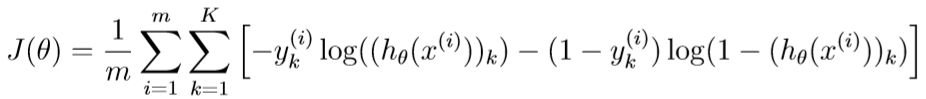在完成了上述的公式代码之后，你可以在屏幕上看见代价为0.287629，下面是这部分的代码
a1 = [ones(m, 1) X];   %5000x401
z2 = a1 * Theta1';     %5000x25   Theta1 25*401
a2 = sigmoid(z2);      %5000x25
a2 = [ones(m, 1) a2];  %5000x26
z3 = a2 * Theta2';     %5000x10   Theta2 10×26
a3 = sigmoid(z3);      %5000x10
h = a3;                %5000x10

u = eye(num_labels);
y = u(y,:);
J = 1/m*(sum(sum(-y .* log(h) - (1 - y) .* log(1 - h))));

1.4 正则化代价函数
公式如下：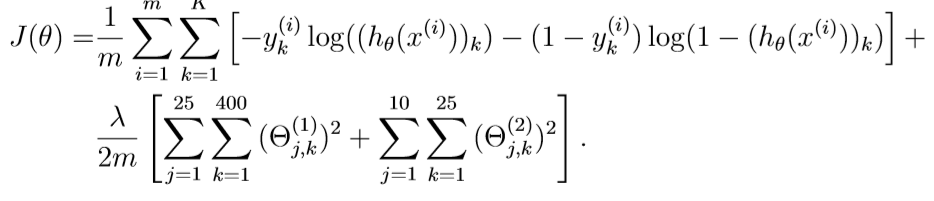这就是在上面的基础上再加上正则项目，但是要注意这个正则项是从1开始计算的。
修改这部分代码即可：
regularization = lambda/(2 * m) * (sum(sum(Theta1(:,2:end) .^ 2)) + sum(sum(Theta2(:,2:end) .^ 2)));
J = J + regularization;

这时候的cost就变成了0.383770.
2 后向传播 nnCostFunction.m
在这部分的练习中，你将会用后向传播算法计算神经网络代价函数的梯度。你需要继续完成nnCostFunction.m使得这个函数返回一个合适的梯度值。
2.1 Sigmoid函数的导数 sigmoidGradient.m
为了帮助你开始这个部分的练习，你将要最先实现sigmoid梯度函数，如下：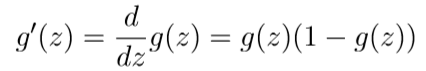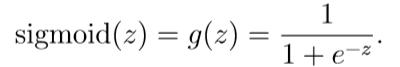根据上述公式完成sigmoidGradient.m函数，当你完成了，你可以通过调用函数来进行验证，当z的值都很大时，不管正负，结果应该是接近于0的，当z=0，其值应为0.25。你的公式不仅要适用于一个数字，对于向量矩阵都能计算出每一个元素的值。
function g = sigmoidGradient(z)
%SIGMOIDGRADIENT returns the gradient of the sigmoid function
%evaluated at z
%   g = SIGMOIDGRADIENT(z) computes the gradient of the sigmoid function
%   evaluated at z. This should work regardless if z is a matrix or a
%   vector. In particular, if z is a vector or matrix, you should return
%   the gradient for each element.
% ====================== YOUR CODE HERE ======================
% Instructions: Compute the gradient of the sigmoid function evaluated at
%               each value of z (z can be a matrix, vector or scalar).
s = 1.0 ./ (1.0 + exp(-z));
g = s .* (1-s);
% =============================================================
end

2.2 随机初始化 randInitializeWeights.m
当训练神经网络时，随机的初始化参数来防止对称现象的发生。一个有效的随机初始化的方法是，随机地在[−ε init,init]初始化Θ的值。代码如下：
function W = randInitializeWeights(L_in, L_out)
W = zeros(L_out, 1 + L_in);
epsilon_init = 0.12;
W = rand(L_out, 1 + L_in) * 2 * epsilon_init - epsilon_init;
end

2.3 后向传播
给出训练样本，我们想要实现前向传播去计算所有的在这个神经网络里面的激活单元，包括输出层的假设函数值。然后，对于每一个l的每一个结点，我们要计算偏差项δ。
对于输出结点，我们会直接计算不同的神经网络激活单元和正确的目标值。而对于隐藏层，你将通过第l+1层的节点偏差项的权重平均值计算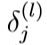。
更详细的，下面是实现后向传播的算法。你需要实现步骤1-4在一个循坏里面，一次处理一个样本。具体来说，你需要实现for循坏，用for i = 1:m然后讲步骤1-4放在这个循环里面，那么第i次迭代就是计算第i个样本。然后第五步会将所有的梯度值相加在除m，获得了神经网络的代价函数的梯度。

将第输入层的值设置成第t个训练样本。先进行前向传播，计算出第2层和第3层的激活单元值。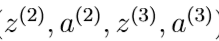在第2层和第3层添加偏置单元。a 1 = [1 ; a 1]
对于每一个输出单元k，设置：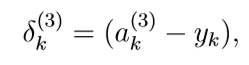这里的yk是0，1变量，就是说当属于这类是就是1，其他的就是0.
3.对于第2层，设置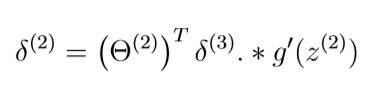4.将梯度值相加。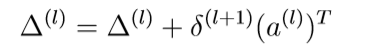5.将梯度值/m。
代码如下：

delta3 = a3 - y;                        % 5000 * 10
delta2 = delta3 * Theta2;               % 5000 * 26
delta2 = delta2(:,2:end);               % 5000 * 25
delta2 = delta2 .* sigmoidGradient(z2); % 5000 * 25

Delta1 = zeros(size(Theta1));           % 25 * 401
Delta2 = zeros(size(Theta2));           % 10 * 26
Delta1 = Delta1 + delta2' * a1;         % 25 * 401 5000×25' * 5000x401
Delta2 = Delta2 + delta3' * a2;         % 10 * 26  5000×10' * 5000x26


梯度检查
在你的神经网络中，你在最小化代价函数J。为了进行梯度检查，你可以想象展开系数Θ1和Θ2，变成一个长向量θ。通过这样做，你可以认为下面的梯度检查过程来代替代价函数。
假设你有一个函数，并且你想要算偏导数，并且你想要检车所得到的结果是不是正确。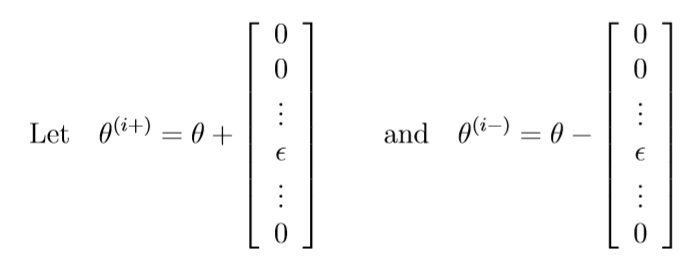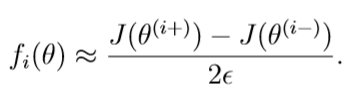这两个值彼此近似的程度取决于J的细节。但是，假设= 10−4，通常会发现上述内容的左边和右边至少要4个有效数字（并且通常还要更多）。在这个部分代码已经写好了，你需要看一看并理解他是怎么样运行的。如果说你的代码正确的话，你将会看到相关误差小于1e-9.
2.5 正则化神经网络
在你成功的完成后向传播算法后，你将要加入正则项。结果表明你可以用后向传播计算完梯度后，再将正则项加进去。公式如下：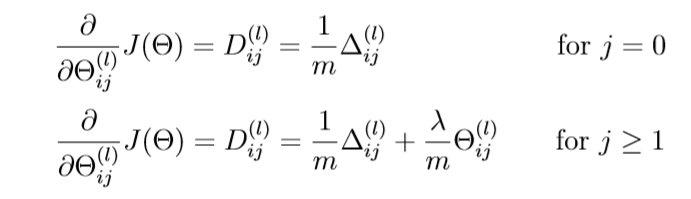要记住偏置单元是不需要被正则的。
2.6 使用fmincg来学习参数
当你成功的完成了上述的步骤之后，下一步是使用fmincg函数去优化参数。完成训练之后，ex.m脚本会向你报告你分类的准确率。如果说你的程序是正确的话，你就会看见95.3%的正确率（这个可能会有1%的变化因为随机初始化参数的不同）。也会随着迭代的增多，正确率会变高。我们建议你去尝试更多迭代次数的神经网络，比如400次等等，也可以尝试改变正则化系数λ。通过正确的学习设置，让神经网络完美的拟合训练集是很有可能的。
3 隐层可视化
一种理解你的神经网络的方法是学习可视化隐层神经元所代表的。通俗一点，给出一个特殊的隐层单元，将他所计算的东西可视化的一个方法是找一个能激活他的输入x。
总结
对于神经网络这节，是比较抽象难理解的一个章节。包括做题目的时候按照老师说的新手用循坏来做。发现其实还是矩阵来做更加清晰。当然，对于这些章节还是需要再反复斟酌的。对于这篇文章还是比较乱，在我的草稿箱里存了好久，仅供参考！下面是我的成绩：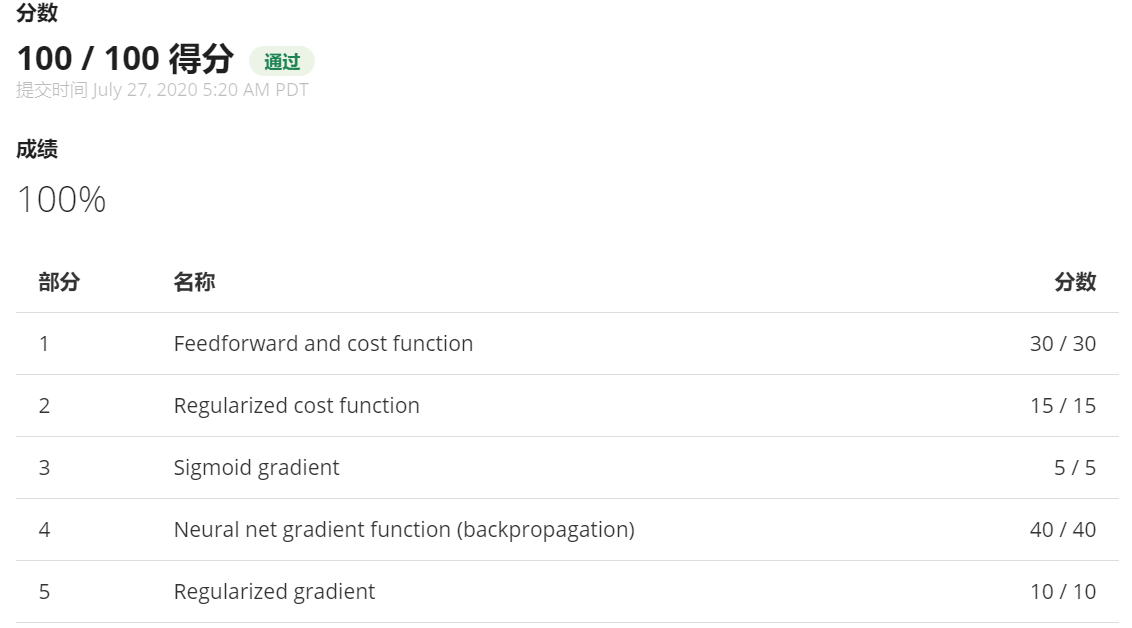展开全文神经网络
• 这篇文章是基于bilibili的吴恩达机器学习经典名课【中英字幕】所写的第三周的编程练习，这个作业是关于逻辑回归的。 作业大纲1 多分类问题1.1 数据集1.2 数据可视化1.3 向量化逻辑回归 1 多分类问题 在这个任务中，...
这篇文章是基于bilibili的吴恩达机器学习经典名课【中英字幕】所写的第三周的编程练习，这个作业是关于逻辑回归的。
作业大纲1 多分类问题1.1 数据集1.2 数据可视化1.3 向量化逻辑回归 lrCostFunction1.3.1 向量化代价函数1.3.2 向量化梯度1.3.3 向量化正则逻辑函数1.4 一对多分类 oneVsAll.m1.4.1 一对多预测 predictOneVsAll.m2 神经网络2.1 模型表示2.2 前馈传播和预测  predict.m总结
1 多分类问题
在这个任务中，你需要使用逻辑回归和神经网络去解决数字识别问题（从0-9）。
1.1 数据集
老师给了ex3data1.mat这个文件，文件中包含了5000个手写的数字训练集。每一个训练集是20px*20px的灰度图像，并且这个图像被展开形成一个400维的向量。值得注意的是，为了兼容matlab的下标，将0写成10，其余的不变。
1.2 数据可视化
在这个部分，我们将调用 displayData function去随机展示100个数字，如下图：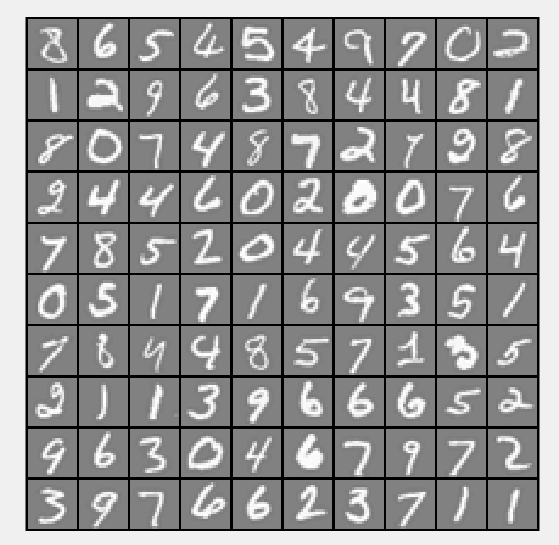1.3 向量化逻辑回归 lrCostFunction
你需要使用多次的一对多逻辑回归模型建立多元分类器。因为有十分类，所以需要训练十个独立的逻辑回归分类器。为了让训练更有效率，确保你的代码向量化是非常重要的。在这个部分里面，你需要使用实现逻辑回归的向量化版本，不能使用任何的循环。
1.3.1 向量化代价函数
这个是不带正则项的代价函数。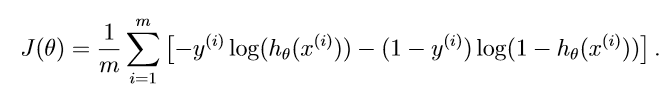1.3.2 向量化梯度
不带正则项的梯度函数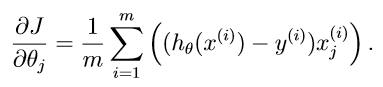1.3.3 向量化正则逻辑函数
这个是带正则项的代价函数。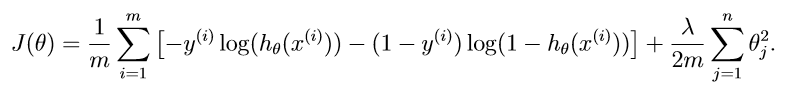带正则项的梯度函数，需要注意的是对于j=0的时候我们不需要计算他的正则项。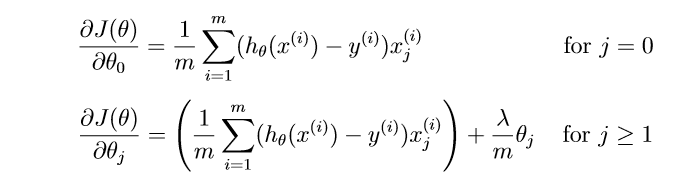代码如下：
function [J, grad] = lrCostFunction(theta, X, y, lambda)
m = length(y); % number of training examples
h = sigmoid(X*theta);
temp = theta;
temp(1) = 0;  % 正则化时theta0没有在求和多项式中
J =  -1/m * (y'*log(h)+(1-y)'*log(1-h)) + lambda/(2*m) * (temp'*temp);
grad = 1/m * (X'*(h - y)) + lambda/m*temp;

1.4 一对多分类 oneVsAll.m
在这个练习部分中，你将通过训练多元正则逻辑回归分类器来实现一对多分类。在手写数字训练集中，你将要实现十个分类器。
function [all_theta] = oneVsAll(X, y, num_labels, lambda)
m = size(X, 1);
n = size(X, 2);
all_theta = zeros(num_labels, n + 1);
X = [ones(m, 1) X];
for c = 1:num_labels
initial_theta = zeros(n + 1, 1);
options = optimset('GradObj', 'on', 'MaxIter', 50);
all_theta(c,:) = fmincg (@(t)(lrCostFunction(t, X, (y == c), lambda)),initial_theta, options);
end

1.4.1 一对多预测 predictOneVsAll.m
在完成训练之后，就可以用得到的模型进行训练啦，对于每一个输入，你应该计算每一个类别的可能性。完成predictOneVsAll.m的编程，将模型的准确率输出。(准确率是94.9%)
function p = predictOneVsAll(all_theta, X)
m = size(X, 1);
num_labels = size(all_theta, 1);
p = zeros(size(X, 1), 1);
X = [ones(m, 1) X];
type = sigmoid(X*all_theta');
for i =1:m
A = type(i,1:num_labels);
p(i) = find(A==max(A));
end
end2 神经网络
逻辑回归不能组成更复杂的假设，因为它是一个线性分类器，在这个部分的练习中，你将实现神经网络去识别手写数字。这周的神经网络的参数我们已经训练好，你需要实现的是编写前馈传播算法去进行识别。
2.1 模型表示
本次训练的模型是一个三层的神经网络，一个输入层，有400个单元。一个隐含层，有25个单元。一个输出层，有十个单元，分别对应了十个数字类别。
2.2 前馈传播和预测  predict.m
function p = predict(Theta1, Theta2, X)
%PREDICT Predict the label of an input given a trained neural network
%   p = PREDICT(Theta1, Theta2, X) outputs the predicted label of X given the
%   trained weights of a neural network (Theta1, Theta2)
% Useful values
m = size(X, 1);
num_labels = size(Theta2, 1);
X = [ones(m,1),X];
% You need to return the following variables correctly
p = zeros(size(X, 1), 1);

% ====================== YOUR CODE HERE ======================
% Instructions: Complete the following code to make predictions using
%               your learned neural network. You should set p to a
%               vector containing labels between 1 to num_labels.
%
% Hint: The max function might come in useful. In particular, the max
%       function can also return the index of the max element, for more
%       information see 'help max'. If your examples are in rows, then, you
%       can use max(A, [], 2) to obtain the max for each row.
%
type1 = sigmoid(X*Theta1');
type1 = [ones(m,1),type1];
type2 = sigmoid(type1*Theta2');
for i =1:m
A = type2(i,1:num_labels);
p(i) = find(A==max(A));
end
end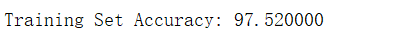总结
以前对于多元的分类问题总是觉得很难，没有办法下手，在看了老师的课之后，就觉得还是蛮有意思的。在这次的练习中也稍微出现了一些些小问题。还是没有把公式了解透彻吧。希望抓紧时间学完机器学习！！！
我的成绩：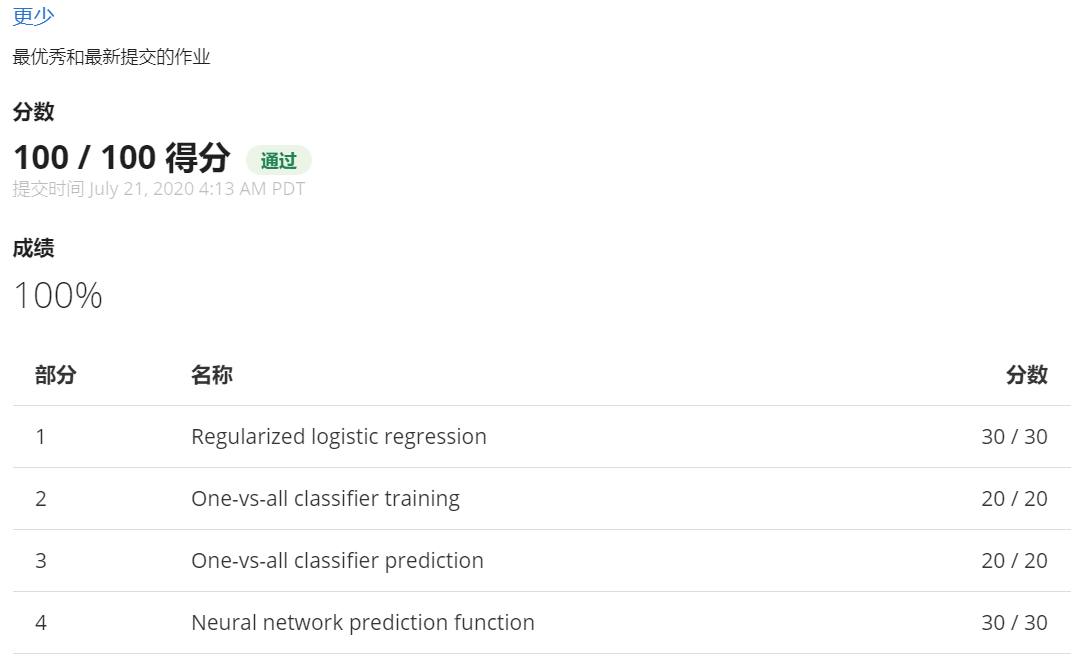展开全文神经网络 逻辑回归
• 本文基于吴恩达老师第六周的练习，在这次的练习中，你将会实现正则化的线性回归并且用它去实现不同的偏差和方差的性质。 作业大纲1 正则化线性回归1.1 数据可视化1.2 正则化线性回归代价函数1.3 正则化线性回归梯度 ...
本文基于吴恩达老师第六周的练习，在这次的练习中，你将会实现正则化的线性回归并且用它去实现不同的偏差和方差的性质。
作业大纲1 正则化线性回归1.1 数据可视化1.2 正则化线性回归代价函数1.3 正则化线性回归梯度 linearRegCostFunction.m1.4 线性回归拟合2 偏差-方差2.1 学习曲线  learningCurve.m3 多项式回归 polyFeatures.m3.1 学习多项式回归3.3 使用交叉验证集来选择λ validationCurve.m我的感想
1 正则化线性回归
在前半部分的练习中，你将实现正则化线性回归通过水库中水位的变化去预测从大坝中流出的水量。在后半部分，你将会通过调试学习算法诊断和测试偏差和方差的影响。
1.1 数据可视化
我们将通过可视化数据集历史水位变化记录x，流出大坝的水量y开始。这个数据集被分为3部分：

训练集
交叉验证集
测试集
画出图形如下：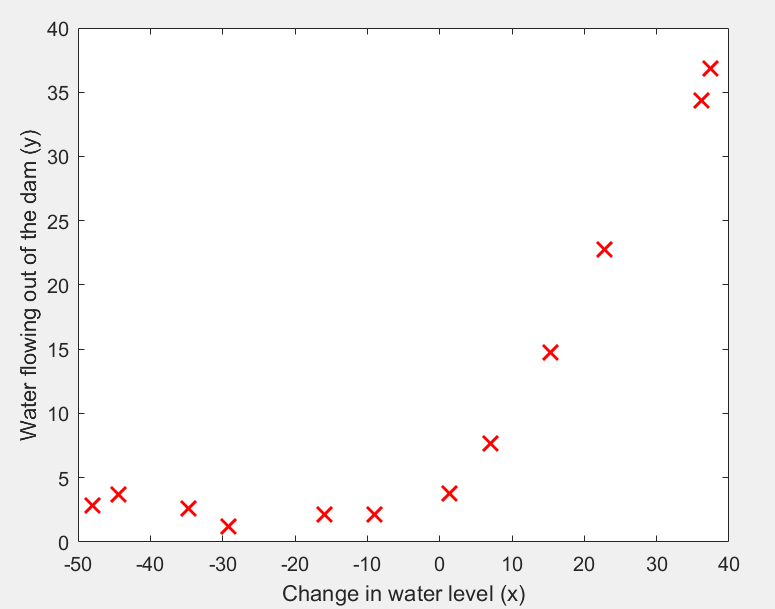在接下来的部分，你将要实现线性回归并且用直线来拟合数据以及画出学习曲线。紧接着
，你会实现多项式回归去找到更好的拟合曲线。

1.2 正则化线性回归代价函数
回想正则化线性回归有以下的代价函数：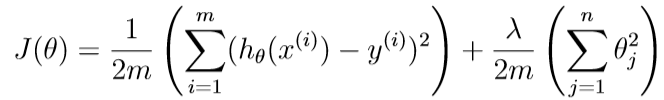其中λ是正则系数，用来控制正则化的程度（防止过拟合）。正则项对总成本J进行惩罚。当模型中θj增加时，惩罚也会加大。记住θ0是不需要正则化的。
现在你需要完成linearRegCostFunction.m。你的任务是完成计算正则化线性回归的代价函数。如果可能，尝试向量化你的代码避免使用循坏。当你完成这部分的内容，你会看到一个结果为303.993。因为这个和下面正则化线性回归梯度的是写在一个脚本文件里面的，因此代码不在赘述。
1.3 正则化线性回归梯度 linearRegCostFunction.m
这里的偏导公式如下：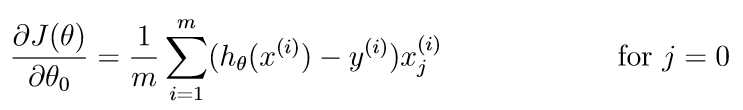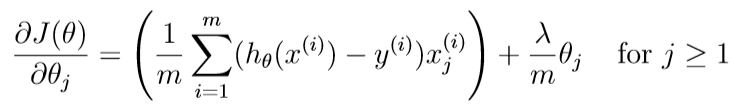在linearRegCostFunction.m添加代码去计算梯度值。完成了之后，你会看见一个期望的梯度为[-15.30; 598.250]。
代码：
function [J, grad] = linearRegCostFunction(X, y, theta, lambda)
%LINEARREGCOSTFUNCTION Compute cost and gradient for regularized linear
%regression with multiple variables
%   [J, grad] = LINEARREGCOSTFUNCTION(X, y, theta, lambda) computes the
%   cost of using theta as the parameter for linear regression to fit the
%   data points in X and y. Returns the cost in J and the gradient in grad

% Initialize some useful values
m = length(y); % number of training examples
% You need to return the following variables correctly
h =  X*theta;
J = (h-y)'*(h-y)/(2*m)+lambda*theta(2:end)'*theta(2:end)/(2*m);
grad = zeros(size(theta));
temp=theta;
temp(1,1)=0;%将第一个值为0
grad=X'*(h-y)/m+lambda*temp/m;
% ====================== YOUR CODE HERE ======================
% Instructions: Compute the cost and gradient of regularized linear
%               regression for a particular choice of theta.
%
%               You should set J to the cost and grad to the gradient.
%

% =========================================================================
grad = grad(:);
end

1.4 线性回归拟合
一旦你的代价函数和梯度能够正确的工作，ex5.m接下来的部分会运行trainLinearReg.m里面的代码去计算最优化的θ值。训练函数会使用fmincg函数优化代价函数。
在这部分，正则系数λ=0.因为我们现在对于线性回归是在2维的θ，正则化不会那么有效在那么低维的情况下。在接下来的练习中，你将会使用正则化多项式回归。
最后，脚本ex5.m也会画出最好的拟合线。结果告诉我们这个模型不能够很好的拟合数据，因为数据是非线性的。当你可视化了最好的拟合是一种可能的方式去判断你的学习算法是否可行，但是这种方法并不总是可行。在下一部分中，你将会实现画出学习曲线来帮助你判断你的学习算法即使可视化数据并不容易。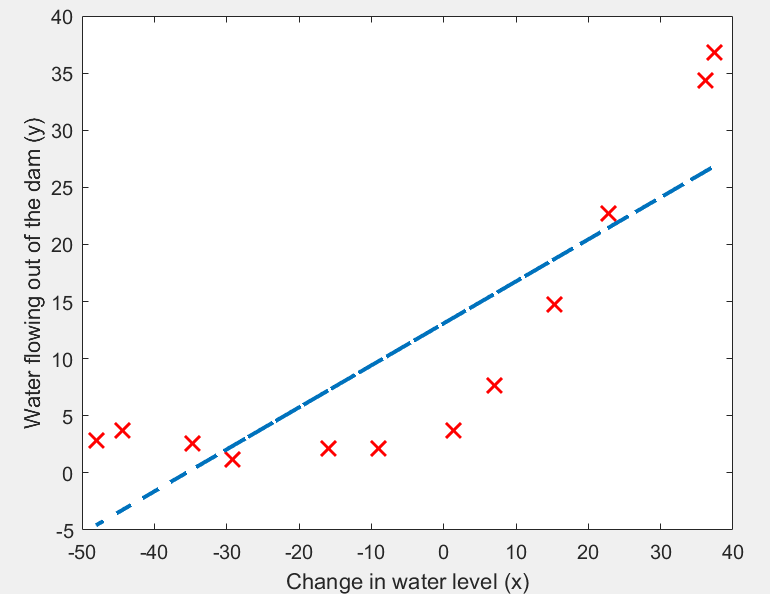2 偏差-方差
机器学习中一个重要的概念就是偏差和方差的比较。高偏差的模型对于数据不够复杂而趋于欠拟合，高方差的模型则会导致在训练集上过拟合。
在这部分的练习中，你将要画出训练误差和测试误差的学习曲线来判断偏差-方差问题。
2.1 学习曲线  learningCurve.m
你现在要完成产生学习曲线的代码，这对你调试你的学习算法会很有用。回想一下，学习曲线将训练和交叉验证误差绘制为训练集大小的函数。你需要完成learningCurve.m，以致于它能够返回一个训练集和交叉验证集的误差向量。
为了画出学习曲线，我们需要对于不同大小的训练集来找到对应的训练和交叉验证集误差。为了获得不同的训练集大小，你应该要使用训练集X的不同的子集。特别地，对于i个大小的训练集，你应该使用 X(1:i,:)和y(1:i)。
你可以使用trainLinearReg函数去找到θ的值，注意λ是learningCurve.m的传入参数，在得到θ的取值之后，你应该计算出训练和交叉验证集的误差。回想训练集的误差是如下定于的：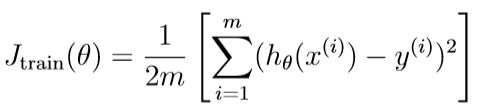特别的，注意训练集误差是不包含正则项的。使用你现有的代价函数并将λ置为0是一种计算训练误差的方法。当你计算训练误差以及交叉验证误差，请你确认它是计算训练子集 (i.e., X(1:n,:) and y(1:n)）而不是整个训练集。但是对于交叉验证误差，你应该计算整个的交叉验证集。并把误差保存在error_train和error_val这两个向量中。
当你完成了，ex5.m会输出如下的学习曲线。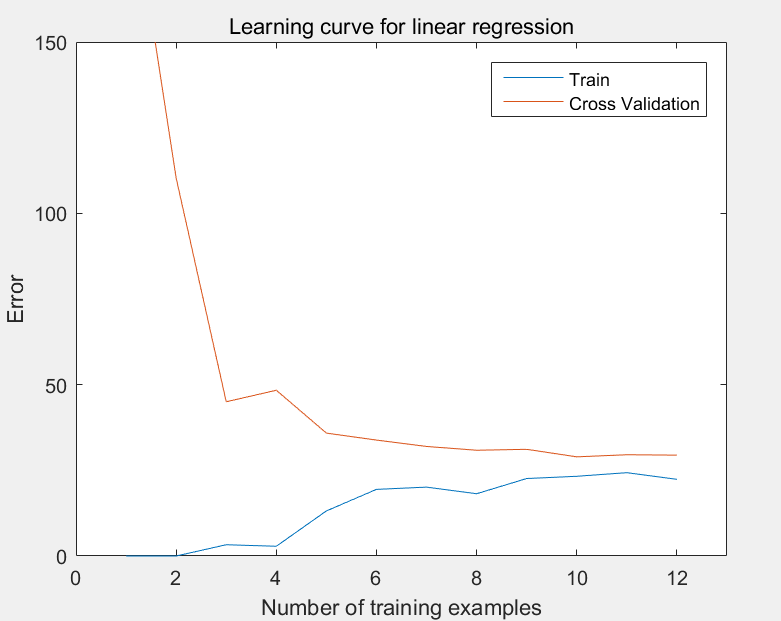观察上图，你可以看到当训练样本变高时交叉验证误差也是高的。这就反映了这个模型中有高偏差的问题，所以说这个线性回归模型过于简单而不能很好地拟合数据。在接下来的部分，你会完成多项式的回归去找到更好的模型来拟合数据集。
代码：
function [error_train, error_val] = ...
learningCurve(X, y, Xval, yval, lambda)
%LEARNINGCURVE Generates the train and cross validation set errors needed
%to plot a learning curve
%   [error_train, error_val] = ...
%       LEARNINGCURVE(X, y, Xval, yval, lambda) returns the train and
%       cross validation set errors for a learning curve. In particular,
%       it returns two vectors of the same length - error_train and
%       error_val. Then, error_train(i) contains the training error for
%       i examples (and similarly for error_val(i)).
%
%   In this function, you will compute the train and test errors for
%   dataset sizes from 1 up to m. In practice, when working with larger
%   datasets, you might want to do this in larger intervals.
%
% Number of training examples
m = size(X, 1);
% You need to return these values correctly
error_train = zeros(m, 1);
error_val   = zeros(m, 1);
% ====================== YOUR CODE HERE ======================
% Instructions: Fill in this function to return training errors in
%               error_train and the cross validation errors in error_val.
%               i.e., error_train(i) and
%               error_val(i) should give you the errors
%               obtained after training on i examples.
%
% Note: You should evaluate the training error on the first i training
%       examples (i.e., X(1:i, :) and y(1:i)).
%
%       For the cross-validation error, you should instead evaluate on
%       the _entire_ cross validation set (Xval and yval).
%
% Note: If you are using your cost function (linearRegCostFunction)
%       to compute the training and cross validation error, you should
%       call the function with the lambda argument set to 0.
%       Do note that you will still need to use lambda when running
%       the training to obtain the theta parameters.
%
% Hint: You can loop over the examples with the following:
%
%       for i = 1:m
%           % Compute train/cross validation errors using training examples
%           % X(1:i, :) and y(1:i), storing the result in
%           % error_train(i) and error_val(i)
%           ....
%
%       end
%
% ---------------------- Sample Solution ----------------------
for i = 1:m
Xtr = X(1:i, :);
ytr = y(1:i);
theta = trainLinearReg(Xtr, ytr, lambda);
[error_train(i),grad1] = linearRegCostFunction(Xtr, ytr, theta, lambda);
[error_val(i),grad2] = linearRegCostFunction(Xval, yval, theta, lambda);
end
% -------------------------------------------------------------
% =========================================================================
end

以上是我第一次写的代码，图都能画出来但是不对，这部分没有得分，去网上百度了找到了下面的方法，有分，但是我觉得没什么差别，有大佬做到的话可以告诉我为什么：
for i = 1:m
theta = trainLinearReg(X(1:i,:),y(1:i),lambda);
error_train(i) = 1/(2*i) * sum((X(1:i,:) * theta - y(1:i)).^2);
error_val(i) = 1/(2*size(Xval,1)) * sum((Xval * theta - yval).^2);
end

3 多项式回归 polyFeatures.m
线性回归模型的问题在对这个训练集过于简单，导致了欠拟合（高偏差）。在这个部分的练习中，你会通过增加更多的特征来解决这个问题。
使用多项式回归的假设如下：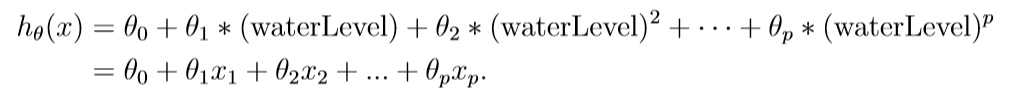通过定义x1=(waterLevel),x2 = (waterLevel)2,…, xp = (waterLevel)p,我们就获得一个有着初始数据(水位)的不同次数的线性回归模型。
现在，你将在数据集中加入更高次数的x。在此节中，你的任务是完成polyFeatures.m里面的函数，舍得这个函数能够映射出训练集m1的原始X的更高次数的数据。特别的，当一个训练集X传入函数，函数应该返回一个mp的矩阵X_poly，第一列是原始X，第二列是X2，第三列是X3，以此类推。注意你不需要增加x0在这个函数中。
现在你有了一个可以映射高维的特征，ex5.m的Part 6会应用这个训练集以及你还未使用过的交叉验证集。
代码：
function [X_poly] = polyFeatures(X, p)
%POLYFEATURES Maps X (1D vector) into the p-th power
%   [X_poly] = POLYFEATURES(X, p) takes a data matrix X (size m x 1) and
%   maps each example into its polynomial features where
%   X_poly(i, :) = [X(i) X(i).^2 X(i).^3 ...  X(i).^p];
%
% You need to return the following variables correctly.
X_poly = zeros(numel(X), p);
% ====================== YOUR CODE HERE ======================
% Instructions: Given a vector X, return a matrix X_poly where the p-th
%               column of X contains the values of X to the p-th power.
%
%
for i=1:p
X_poly(:,i) = X.^i;
end
% =========================================================================
end

3.1 学习多项式回归
完成了上述函数之后，ex5.m会使用你的线性回归代价函数来训练多项式函数。
记住即使我们有了高次的项，我们解决的实际上还是线性回归的优化问题。多项式早就转化成了线性回归的特征。所以我们使用早就写好的代价函数和梯度是没有问题的。
在这部分中的练习中，你将会使用8次多项式。结果表明如果我们直接使用目标数据，并不会很好的起作用因为特征有着糟糕的规模。因此，我们需要特征的归一化。
接着的就不需要再自己动手写代码了。老师在这部分是让我们修改λ的值来观察高方差和高偏差。高偏差在上述说过，就是测试集和交叉验证集的错误都比较高，欠拟合。那么将λ设为0，结果如下：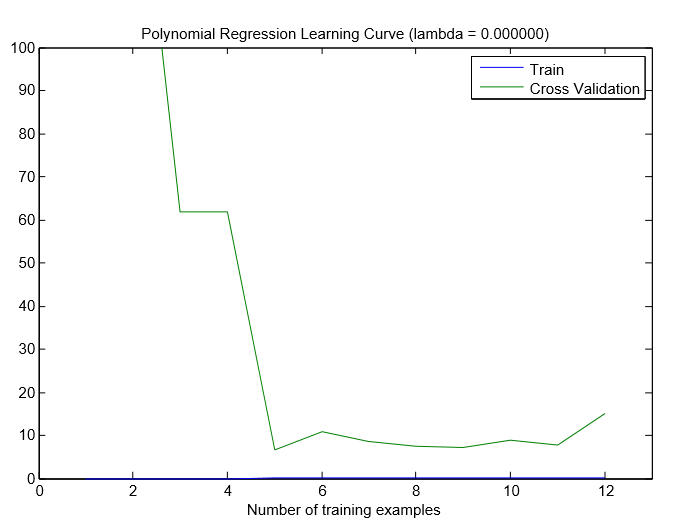我们可以看到训练误差和交叉验证误差有着较大的间隔，并且训练集的误差较低（都快没有了），但是交叉验证集的误差还是比较高，这就是过拟合，高方差的问题了。
接着是λ=1的图像：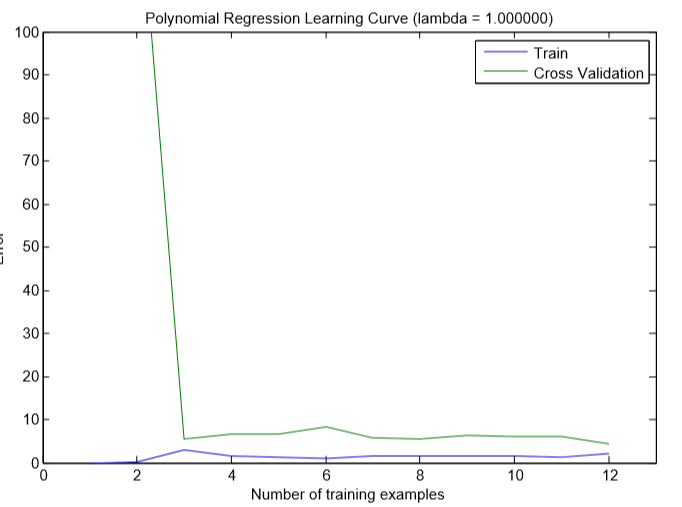可以看出，间隙变小了，并且交叉验证误差也小了。
3.3 使用交叉验证集来选择λ validationCurve.m
在之前的练习中，你已经观察到λ的值可以极大地影响到在训练集和交叉验证集上的正则化多项式回归。特别地，一个没有经过正则化的模型可以很好地拟合训练集，但是泛化能力很差。反过来，过大的λ值并不能很好的拟合训练集和测试集。对于λ的适当选择可以较好的拟合数据。
在这节中，你会实现一种自动选择λ的方法。更具体的，你会使用交叉验证集去评估那个λ是比较好的。在使用了交叉验证集选择了最好的λ之后，我们可以通过在测试集上评估模型，评价他在看不见的数据上的表现会有多好。
你的任务是完成validationCurve.m的代码。特别的，你应该需要使用trainLinearReg 函数通过λ的不同取值来训练模型，然后计算训练误差以及交叉验证集误差。你应该在下列的范围内取λ的值 {0,0.001,0.003,0.01,0.03,0.1,0.3,1,3,10}。
function [lambda_vec, error_train, error_val] = ...
validationCurve(X, y, Xval, yval)
%VALIDATIONCURVE Generate the train and validation errors needed to
%plot a validation curve that we can use to select lambda
%   [lambda_vec, error_train, error_val] = ...
%       VALIDATIONCURVE(X, y, Xval, yval) returns the train
%       and validation errors (in error_train, error_val)
%       for different values of lambda. You are given the training set (X,
%       y) and validation set (Xval, yval).
%
% Selected values of lambda (you should not change this)
lambda_vec = [0 0.001 0.003 0.01 0.03 0.1 0.3 1 3 10]';
% You need to return these variables correctly.
error_train = zeros(length(lambda_vec), 1);
error_val = zeros(length(lambda_vec), 1);
% ====================== YOUR CODE HERE ======================
% Instructions: Fill in this function to return training errors in
%               error_train and the validation errors in error_val. The
%               vector lambda_vec contains the different lambda parameters
%               to use for each calculation of the errors, i.e,
%               error_train(i), and error_val(i) should give
%               you the errors obtained after training with
%               lambda = lambda_vec(i)
%
% Note: You can loop over lambda_vec with the following:
%
%       for i = 1:length(lambda_vec)
%           lambda = lambda_vec(i);
%           % Compute train / val errors when training linear
%           % regression with regularization parameter lambda
%           % You should store the result in error_train(i)
%           % and error_val(i)
%           ....
%
%       end
%
%
m=size(X,1);
for i=1:length(lambda_vec),
lambda=lambda_vec(i);
theta = trainLinearReg(X, y, lambda);
error_train(i)=sum((X*theta-y).^2)/2/m;
error_val(i)=sum((Xval*theta-yval).^2)/2/m;
end
% =========================================================================
end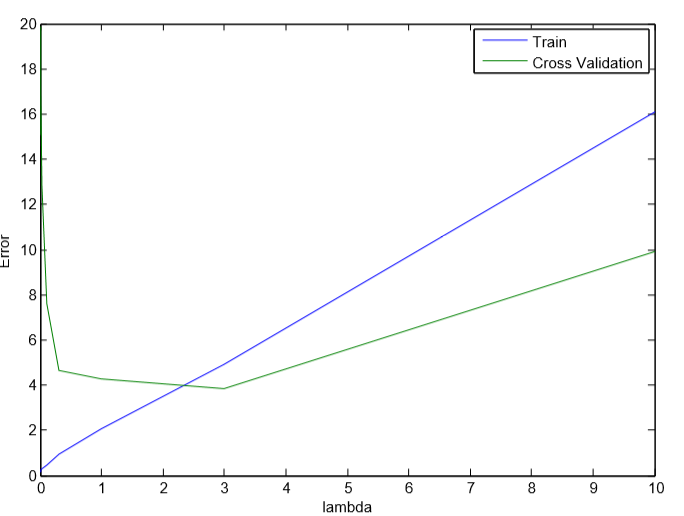从上图可以看出，λ的最佳取值应该是在3左右，因为训练集和交叉验证集的随机划分，交叉验证的误差有时候会比训练集要低。
我的感想
老师讲的课真的是很不错！强推！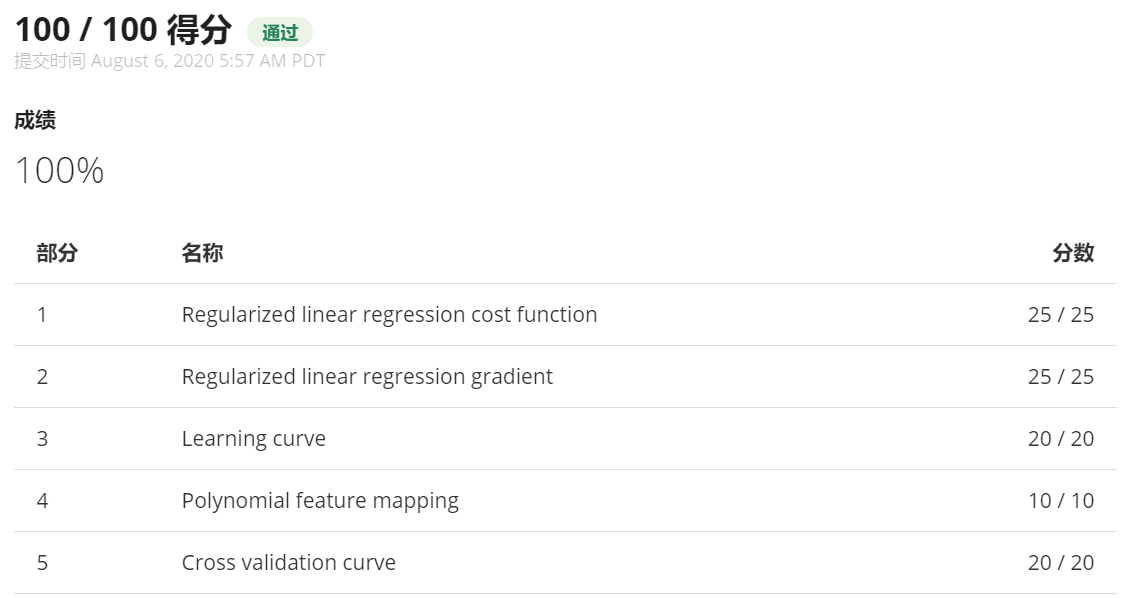展开全文算法
• 吴恩达机器学习ex2-Matlab实现：内含算法代码、数据集、ex2描述文档。
• 吴恩达机器学习ex1-Matlab实现：内含算法代码、数据集、ex1描述文档。
• 吴恩达机器学习Matlab和python源码。。。。。。。。。。。
• 解决matlab2019b吴恩达机器学习提交失败的问题matlab2019b问题复现问题没完这样就完了？大功告成 matlab2019b 之前在网上看到2019对深度学习方向（神经网络）有相应的功能优化，又碰巧系统盘不够了就重装了matlab，...
• 吴恩达机器学习与深度学习课后代码matlab实现，一元现行回归、多元线性回归、逻辑回归代码
• 吴恩达机器学习（三）—— ex1：Linear Regression（MATLAB+Python） 吴恩达机器学习（四）—— Logisitic回归 吴恩达机器学习（五）—— 正则化 吴恩达机器学习（六）—— ex2：Logistic Regression（MATLAB+Python...
• 吴恩达机器学习作业1-logistic regression。matlab代码
• 吴恩达的cousera机器学习课程课后编程作业，这门课必须实践才能较好的理解
• 也是吴恩达机器学习第6章Octave/Matlab 教程中讲解用的基础程序 5+6 2^6 1==2 1~=2 1&&0 1||0 xor(0,1) a = pi; disp (sprintf ('2 decimals : %0.2f ' , a )) disp (sprintf ('6 decimals : %...
• 吴恩达机器学习作业1-linear regression。matlab代码。
• 吴恩达机器学习课后练习题代码，以及相应的分析，用MATLAB编写，欢迎下载
• 机器学习matlab操作基础1、基本操作2、移动数据3、计算数据4、绘图数据5、控制语句：for，while，if语句6、向量化 1、基本操作 参考视频: 5 - 1 - Basic Operations (14 min).mkv 打开matlab(R2019b) 这是 matlab...
• coursera吴恩达机器学习课程作业自写Python版本+Matlab原版
• Coursera上吴恩达老师的机器学习课程作业machine-learning-ex1~ex8代码(matlab)
• matlab中m文件编辑器不同部分有不同的颜色，如下图，采用的是cell模式：用两个%%加空格，表示一个新的Cell段。下图“%% Mach...”,直到出现下一个“%% ....”，是一个Cell。有了代码Cell后，可以只运行M文件中某个......

# matlab吴恩达机器学习matlab 订阅